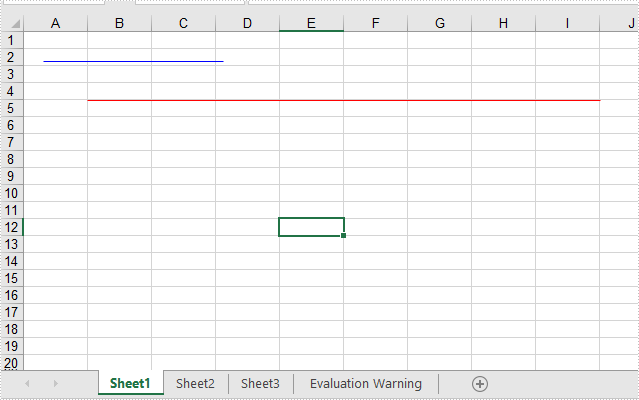# C#/VB.NET Add lines to Excel worksheets through two points

This article will show you how to add lines to Excel worksheets through two points. We could set the point’s location via relative location and Absolute location in pixels.

C#
```using Spire.Xls;
using System.Drawing;

namespace Word
{
class Program
{
static void Main(string[] args)

{
//Initiate a Workbook object and get the first worksheet
Workbook workbook = new Workbook();
Worksheet worksheet = workbook.Worksheets;
//Add a line with relative location
XlsLineShape line = worksheet.TypedLines.AddLine() as XlsLineShape;

//set the column index of the starting point
line.LeftColumn = 2;
line.LeftColumnOffset = 2;
line.TopRow = 5;
line.TopRowOffset = 10;

//set the column index of the end point
line.RightColumn = 10;
line.RightColumnOffset = 10;
line.BottomRow =5;
line.BottomRowOffset = 10;

//Set the color
line.Color = Color.Red;

//Add a line with Absolute location in pixels
XlsLineShape line1 = worksheet.TypedLines.AddLine() as XlsLineShape;
//Set the start point and end point
line1.StartPoint = new Point(20, 30);
line1.EndPoint = new Point(200, 30);

//Set the color
line1.Color = Color.Blue;

workbook.Dispose();
}
}
}
```
VB.NET
```Imports Spire.Xls
Imports System.Drawing

Namespace Word

Class Program

Private Shared Sub Main(ByVal args() As String)
'Initiate a Workbook object and get the first worksheet
Dim workbook As Workbook = New Workbook
Dim worksheet As Worksheet = workbook.Worksheets(0)
'Add a line with relative location
Dim line As XlsLineShape = CType(worksheet.TypedLines.AddLine,XlsLineShape)
'set the column index of the starting point
line.LeftColumn = 2
line.LeftColumnOffset = 2
line.TopRow = 5
line.TopRowOffset = 10
'set the column index of the end point
line.RightColumn = 10
line.RightColumnOffset = 10
line.BottomRow = 5
line.BottomRowOffset = 10
'Set the color
line.Color = Color.Red
'Add a line with Absolute location in pixels
Dim line1 As XlsLineShape = CType(worksheet.TypedLines.AddLine,XlsLineShape)
'Set the start point and end point
line1.StartPoint = New Point(20, 30)
line1.EndPoint = New Point(200, 30)
'Set the color
line1.Color = Color.Blue